# Gronwall summation method

(diff) ← Older revision | Latest revision (diff) | Newer revision → (diff)

A method for summing series of numbers or functions, defined by specifying two functionsandsatisfying certain conditions. A seriescan be summed by the Gronwall method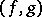to a sumif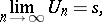where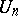,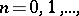is defined by the expansion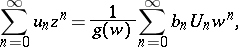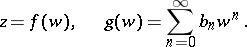The method was introduced by T.H. Gronwall  as a generalization of the de la Vallée-Poussin summation method, to which it is converted byIfthen the Gronwall summation method becomes one of the Cesàro summation methods.

How to Cite This Entry:
Gronwall summation method. Encyclopedia of Mathematics. URL: http://encyclopediaofmath.org/index.php?title=Gronwall_summation_method&oldid=12127
This article was adapted from an original article by I.I. Volkov (originator), which appeared in Encyclopedia of Mathematics - ISBN 1402006098. See original article